# Introduction: Deep Reinforcement Learning

2022.04.14: AlphaGo施工完成.

# Introduction: Deep Reinforcement Learning

## Terminology

Reinforcement Learning(RL)旨在通过智能体环境的不断交互, 教会智能体如何执行任务, 比如让人工智障自己学会玩超级玛丽.

RL相关的术语很多, 下面先在超级玛丽这一游戏场景下介绍RL里面的相关概念.

• Agent(智能体): 强化学习的主体. 即玛丽奥.
• State(状态): 环境的状态, 记为$s \in \mathcal{S}$, 可以理解成超级玛丽的游戏画面.
• Action(动作): 智能体基于当前环境状态可以做出的行为. 记为$a \in \mathcal{A}$. 例如玛丽奥可以向上, 左右跑动.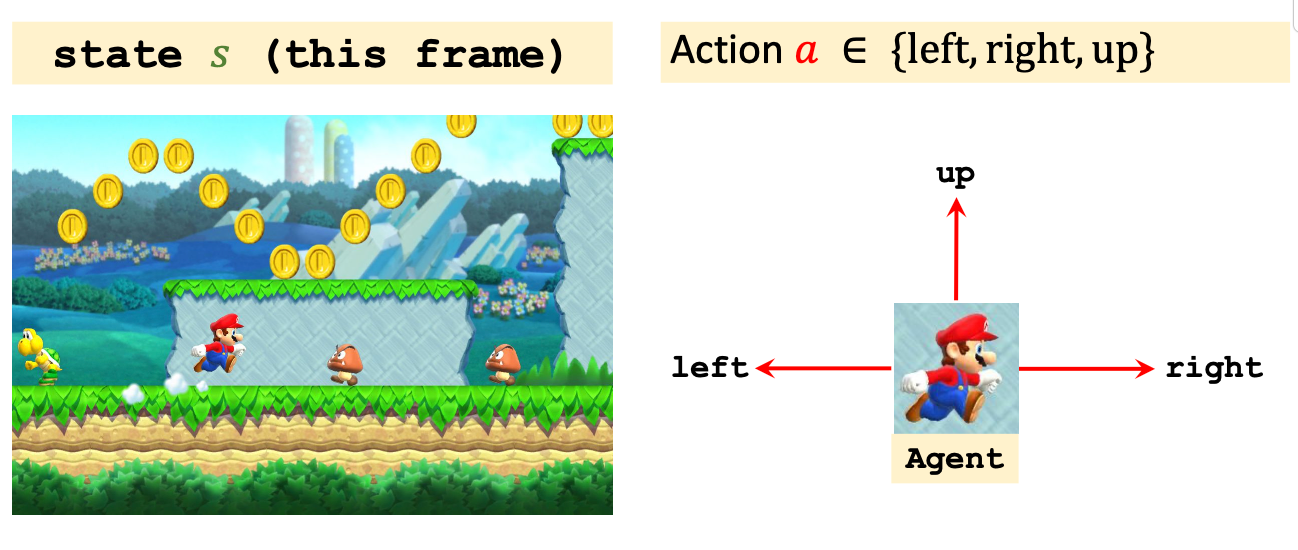• Policy(策略): Agent应该根据状态做出什么决策, 其数学描述个概率密度函数(在这里说的不严谨, 因为例子是一个离散的):
$$\pi(a \mid s)=\mathbb{P}(A=a \mid S=s)$$
根据给出的状态$s$, 都能根据$\pi(a\mid s)$给出一个做出动作$a$ 的概率. 例如在当前超级玛丽画面下, 策略函数可以判断出玛丽奥应该向上走, 还是向左向右走.

为什么要让策略$\pi$ 是随机的? 我认为有两个原因:

1. 因为在与环境的交互中, 如果Agent一直保持恒定的策略运作, 很大概率会被不断变化的环境所击垮. 在后面也可以发现, 在强化学习中, Agent和环境始终保持博弈, 所以才要让Agent的策略变化多端.
2. 鼓励Agent做一些随机动作, 不断探索做出不同动作对环境的影响. 尤其是在Agent训练早期, 随机性应该设置的更大一些.
• Reward(奖励): Agent做出动作后, 给予Agent的奖励, 记为$R$. 其定义方法不唯一. 例如打赢超级玛丽可以定义非常多的奖励, 而吃金币只能获得少量奖励, 被打死要给予负的奖励.

• State Transition(状态转移): 环境因Agent做出了某个动作后从旧状态变为新状态的过程. 可以是确定的也可以是, 通常认为是随机的, 状态转移的随机性来自于环境. 例如玛丽奥的敌人可能向左走也可能向右走. 同样可以用概率密度函数来表示:
$$p(s^\prime\mid s, a) = \mathbb{P}(S^\prime=s \mid S=s, A=a)$$
状态转移函数仅有环境自己可知.

• Episode: RL里的epoch不叫epoch, 与之类似的概念叫 episodes, 指智能体从游戏开始到通关或者结束的过程, 也就是玛丽奥直至通关或死亡的过程称为一个Episode. 因为RL对样本数量要求非常高, 所以让Agent玩一个游戏可能需要成千上万把.

### Randomness in Reinforcement Learning

1. Actions have randomness: Policy函数根据State选择动作的时候存在随机性, 动作是根据Policy Function抽样得到的. 它是Agent的随机性.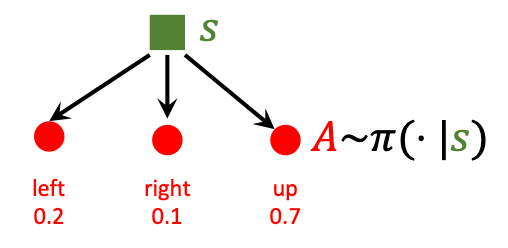2. State transitions have randomness: 状态转移存在随机性, 系统的状态转移也是根据抽样得来的. 它是Environment的随机性.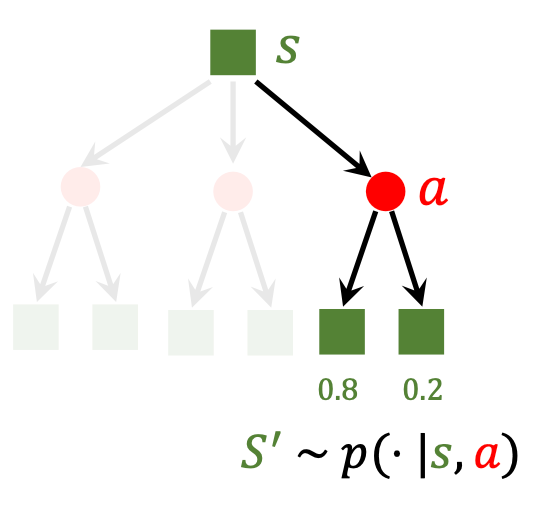### Agent - Environment Interaction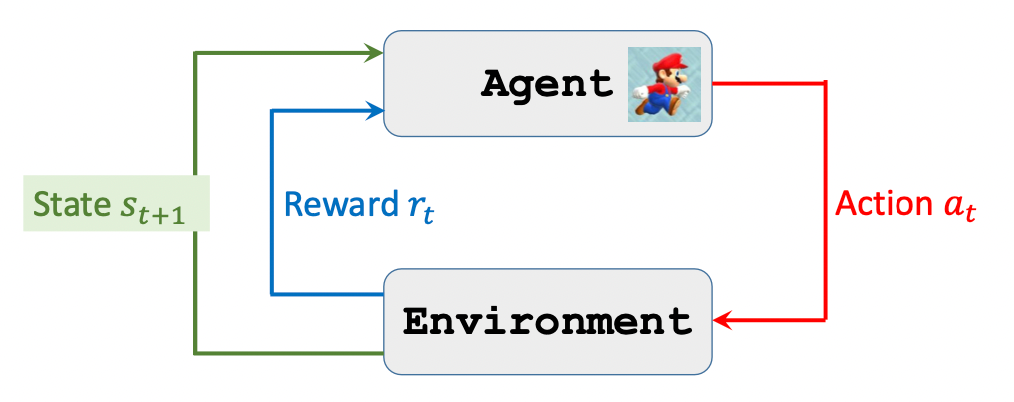Agent总是和环境循环的按照下面的流程交互:

1. 观察环境, 得到状态$s_1$.
2. Agent做出动作$a_1$.
3. 环境状态转移, 得到状态$s_2$, 同时给予Agent奖励$r_1$.
4. Agent做出动作$a_2$.
5. ……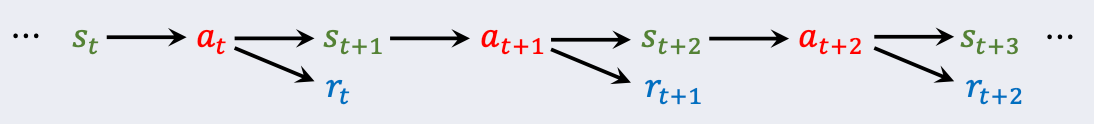$$s_1, a_1,r_1,\ s_2, a_2, r_2,\ \cdots,\ s_T, a_T, r_T.$$
$T$ 为结束时刻.

### Rewards and Returns

#### Return

Return(回报)指的是当前时刻开始到这个episode结束奖励的和, 它的另一个名字是Cumulative Future Reward(未来累计奖励), 所以它和当前时刻的奖励$R_t$ 不是同一个概念:
$$U_t= R_t+ R_{t+1} + R_{t+2} + R_{t+3} + \cdots$$

$$U_t= R_t+ \gamma R_{t+1} + \gamma^2 R_{t+2} + \gamma^3 R_{t+3} + \cdots$$
$\gamma \in [0, 1]$, 叫折扣率, 是个超参. 如果未来和现在的奖励权重相同, 那就令$\gamma=1$, 如果根本不考虑未来的奖励, 那就令$\gamma=0$.

#### Randomness in Returns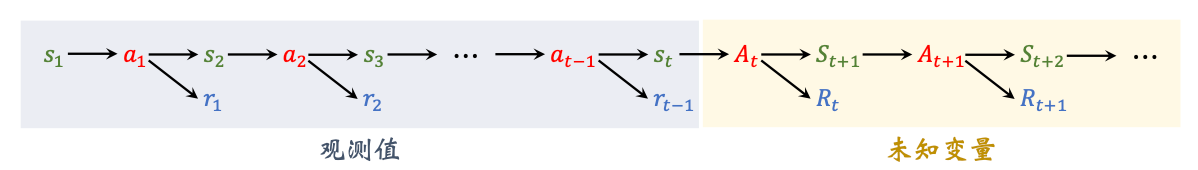### Value Functions

#### Action - Value Function

$U_t$ 取决于$A_t, A_{t+1}, A_{t+2}, \cdots$ 和$S_{t+1}, S_{t+2}, \cdots$, 从$t+1$ 时刻起的所有动作和状态都具有随机性, 如果把$U_t$ 视为$s_t$ 和$a_t$ 的函数, 求条件期望可以去掉随机, 这个函数被称为Action - Value Function(动作价值函数):
$$Q_{\pi}\left(s_{t}, a_{t}\right)=\mathbb{E}\left[U_{t} \mid S_{t}=s_{t}, A_{t}=a_{t}\right]$$

• 动作的概率密度函数是策略函数 $\mathbb{P}(A=a \mid S=s) = \pi(a \mid s)$.
• 状态的概率密度函数是状态转移函数 $\mathbb{P}(S^\prime=s \mid S=s, A=a) = p(s^\prime\mid s, a)$.
• 动作价值函数与$\pi$ 有关. 因为在不同策略函数$\pi$ 下, 条件期望不同.

$$Q^{\star}\left(s_{t}, a_{t}\right)=\max _{\pi} Q_{\pi}\left(s_{t}, a_{t}\right)$$

#### State - Value Function

$$V_{\pi}\left(s_{t}\right) =\mathbb{E}_A\left[Q_{\pi}\left(s_{t}, A\right)\right] =\sum_{a \in \mathcal{A}} \pi\left(a \mid s_{t}\right) \cdot Q_{\pi}\left(s_{t}, a\right)$$

$$V_{\pi}\left(s_{t}\right) =\mathbb{E}_A\left[Q_{\pi}\left(s_{t}, A\right)\right] =\int_{a \in \mathcal{A}} \pi\left(a \mid s_{t}\right) \cdot Q_{\pi}\left(s_{t}, a\right)\mathrm{d}a$$

$V_\pi$ 还能评价$\pi$ 的好坏, 如果$\pi$ 越好, 去掉状态的不确定性(对$S$ 求期望)后的值$\mathbb{E}_S\left[V_{\pi}\left(S\right)\right]$ 就越大.

## Value - Based Reinforcement Learning

### Deep Q - Network

Agent不能变身预言家, 除非… 除非Agent玩了无数把超级玛丽, 对超级玛丽, 那它也和先知没什么区别了, 瞅一眼屏幕就能告诉你要选什么Action.

$$Q(s, a, \mathbf{w}) \approx Q^\star (s, a)$$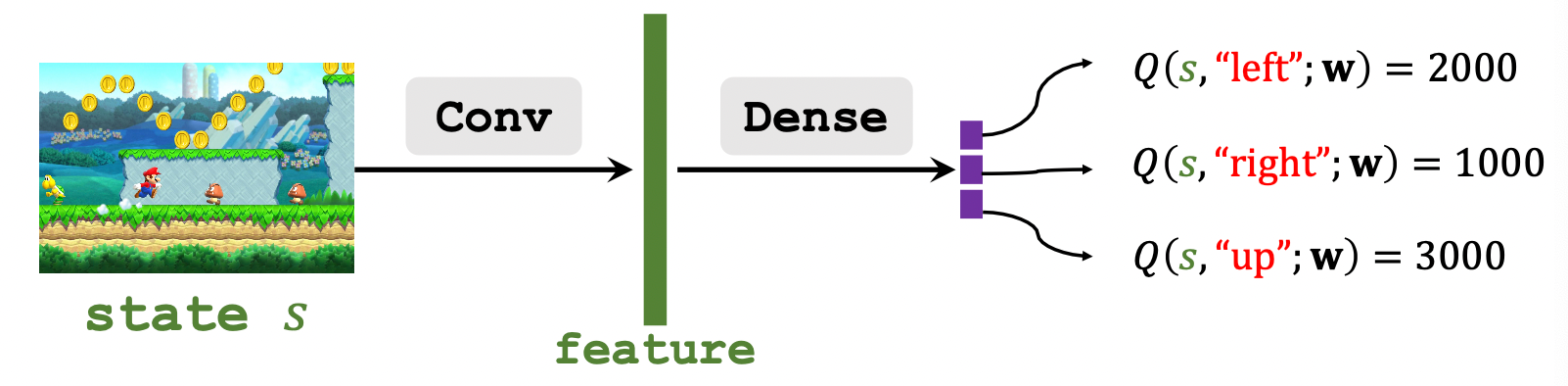1. 观察环境状态$s_t$, 比如用CNN来捕获特征.
2. 用DQN获得Agent各个动作的打分, 让Agent做出打分最大的动作$a_t$.
3. 环境根据$a_t$ 发生状态转移, 新状态为$s_{t+1}$, 同时给予Agent奖励$r_t$.
4. ……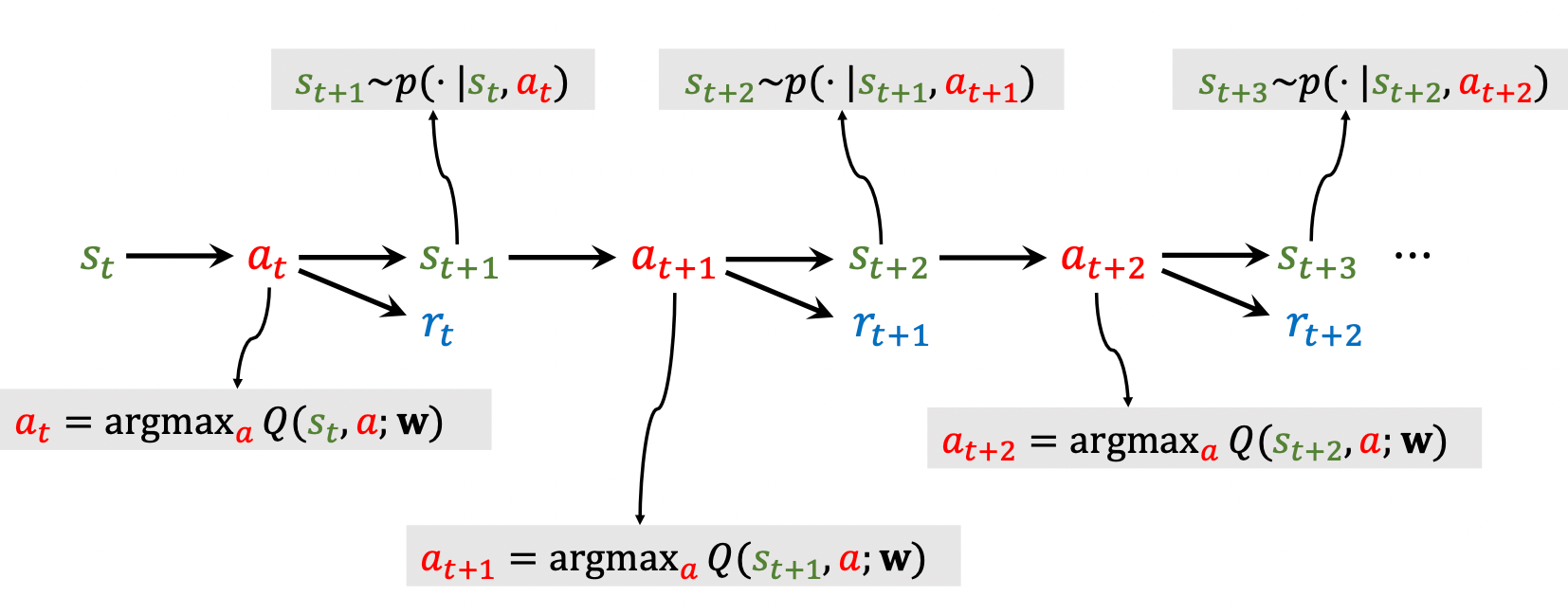### Temporal Difference (TD) Learning

Temporal Differnece(时间差分)算法是训练DQN最常用的方法.

#### Scenario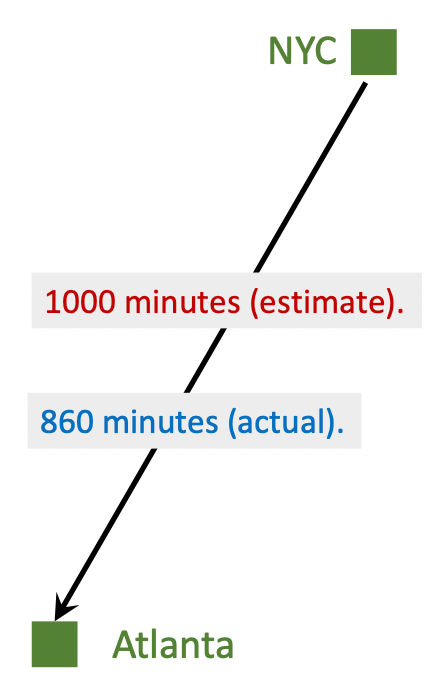• $q=Q(\mathbf{w}), q=1000, y=860$.
• Loss: $L = \frac{1}{2} (q-y)^2$.
• Gradient: $\frac{\partial L}{\partial \mathbf{w}} = \frac{\partial{L}}{\partial{q}} \cdot \frac{\partial{q}}{\partial{\mathbf{w}}} = (q-y)\cdot \frac{\partial Q(\mathbf{w})}{\partial\mathbf{w}}$.
• Gradient Descent: $\mathbf{w}_{t+1} = \mathbf{w}_t - \alpha \cdot \frac{\partial{L}}{\partial\mathbf{w}}|_{\mathbf{w}=\mathbf{w_t}}$.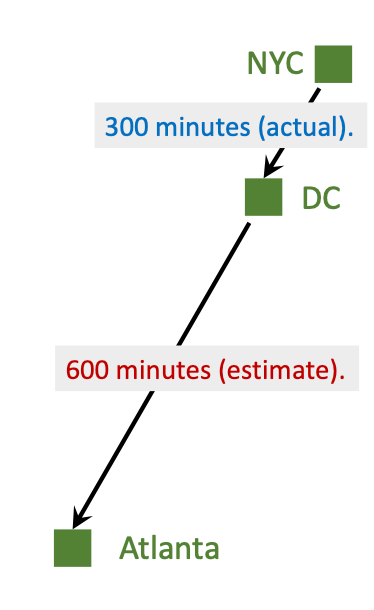• $q=Q(\mathbf{w})=1000, y=300+600=900$.
• Loss: $L = \frac{1}{2}{\left(Q\left(\mathbf{w}\right)-y\right)}^2$.
• Gradient: $\frac{\partial L}{\partial\mathbf{w}} = \frac{\partial{L}}{\partial{q}} \cdot \frac{\partial{q}}{\partial{\mathbf{w}}} = \left(Q\left(\mathbf{w}\right)-y\right)\cdot \frac{\partial Q(\mathbf{w})}{\partial\mathbf{w}}=(1000-900)\cdot\frac{\partial Q(\mathbf{w})}{\partial\mathbf{w}}$.
• Gradient Descent: $\mathbf{w}_{t+1} = \mathbf{w}_t - \alpha \cdot \frac{\partial{L}}{\partial\mathbf{w}}|_{\mathbf{w}=\mathbf{w_t}}$.

$Q(\mathbf{w}) -y = 1000-900=100$ 也称为TD Error $\delta$.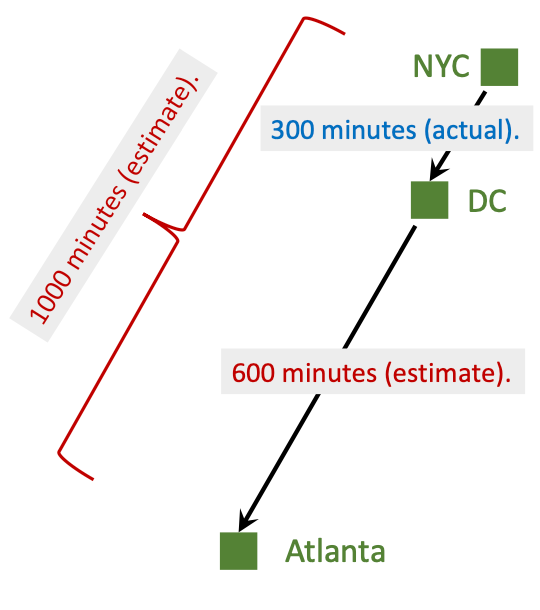#### TD Learning for DQN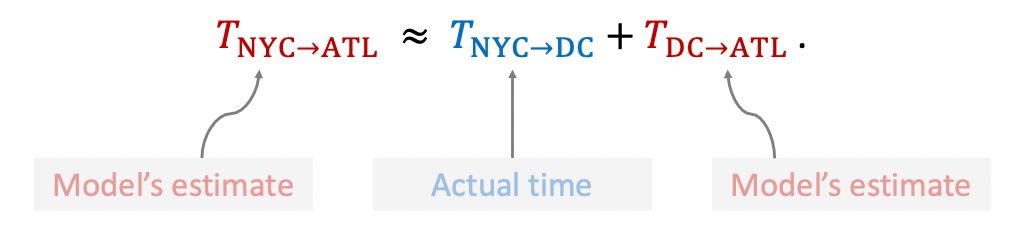$$Q\left(s_{t}, a_{t} ; \mathbf{w}\right) \approx r_{t}+\gamma \cdot Q\left(s_{t+1}, a_{t+1} ; \mathbf{w}\right)$$

\begin{aligned} U_t&= R_t+ \gamma R_{t+1} + \gamma^2 R_{t+2} + \gamma^3 R_{t+3} + \cdots \\ &= R_t+ \gamma \cdot \left(R_{t+1} + \gamma R_{t+2} + \gamma^2 R_{t+3} + \cdots \right) \\ & = R_t + \gamma \cdot U_{t+1} \end{aligned}

$$\mathbb{E}\left[U_t\right] = \mathbb{E} \left[R_t + \gamma \cdot U_{t+1}\right]$$

$$Q(s_t, a_t; \mathbf{w}) \approx \mathbb{E}\left[R_t + \gamma \cdot Q\left(S_{t+1}, A_{t+1}, ; \mathbf{w}\right)\right]$$

• Prediction: $Q(s_t, a_t; \mathbf{w}_t)$.

• TD Target:

在$t+1$ 时刻, 可以观测到奖励$r_t$ 和新的状态$s_{t+1}$, 新的动作$a_{t+1}$ 可以由$Q$ 得到, 故TD Target $y_t$:

\begin{aligned} y_t&= r_t + \gamma \cdot Q(s_{t+1}, a_{t+1} ; \mathbf{w}_t) \\ &= r_t + \gamma \cdot \underset{a}{\max}Q(s_{t+1}, a; \mathbf{w}_t) \end{aligned}

• Loss: $L_t = \frac{1}{2}{[Q(s_t, a_t; \mathbf{w}) - y_t]}^2$.
• Gradient Descent: $\mathbf{w}_{t+1} = \mathbf{w}_t - \alpha \cdot \frac{\partial{L_t} }{\partial\mathbf{w}} |_{\mathbf{w}=\mathbf{w}_t}$.

## Policy - Based Reinforcement Learning

### Policy Network

"神经网络时代的哲学: 难算的我们都用神经网络来拟合!"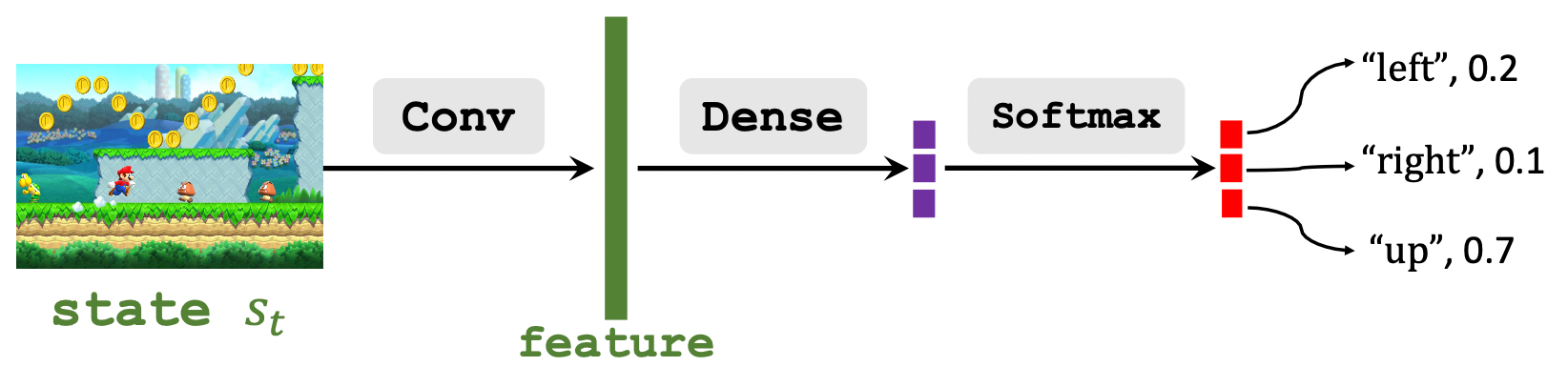### State - Value Function Approximation

#### Policy - Based Reinforcement Learning

$$\displaylines{ V_{\pi}\left(s_{t}\right) =\mathbb{E}_A\left[Q_{\pi}\left(s_{t}, A\right)\right] =\sum_{a \in \mathcal{A}} \pi\left(a \mid s_{t}\right) \cdot Q_{\pi}\left(s_{t}, a\right) \\ \Downarrow \\ V\left(s_{t};\boldsymbol{\theta}\right) =\sum_{a \in \mathcal{A}} \pi\left(a \mid s_{t};\boldsymbol{\theta}\right) \cdot Q_{\pi}\left(s_{t}, a\right) }$$

$$J(\boldsymbol{\theta})=\mathbb{E}_{S}[V(S ; \boldsymbol{\theta})]$$

$$\boldsymbol{\theta} \leftarrow \boldsymbol{\theta}+\beta \cdot \frac{\partial V(s ; \boldsymbol{\theta})}{\partial\boldsymbol{\theta}}$$
$\beta$ 为学习率. 但这里的梯度指的是策略梯度.

\begin{aligned} \frac{\partial V(s ; \boldsymbol{\theta})}{\partial \boldsymbol{\theta}} &=\frac{\partial \sum_{a} \pi(a \mid s ; \boldsymbol{\theta}) \cdot Q_{\pi}(s, a)}{\partial \boldsymbol{\theta}} \\ &=\sum_{a} \frac{\partial \pi(a \mid s ; \boldsymbol{\theta}) \cdot Q_{\pi}(s, a)}{\partial \boldsymbol{\theta}} \\ &=\sum_{a} \frac{\partial \pi(a \mid s ; \boldsymbol{\theta})}{\partial \boldsymbol{\theta}} \cdot Q_{\pi}(s, a) \end{aligned}

\begin{aligned} \frac{\partial V(s ; \boldsymbol{\theta})}{\partial \boldsymbol{\theta}} &=\sum_{a} \frac{\partial \pi(a \mid s ; \boldsymbol{\theta})}{\partial \boldsymbol{\theta}} \cdot Q_{\pi}(s, a) \\ &=\sum_{a} \pi(a \mid s ; \boldsymbol{\theta}) \cdot \frac{\partial \log \pi(a \mid s ; \boldsymbol{\theta})}{\partial \boldsymbol{\theta}} \cdot Q_{\pi}(s, a) \\ &= \mathbb{E}_A\left[ \frac{\partial{\log \pi(A \mid s ; \boldsymbol{\theta})}}{\partial \boldsymbol{\theta}} \cdot Q_{\pi}(s, A)\right] \end{aligned}

$$\frac{\partial \log \left[\pi(\boldsymbol{\theta})\right]}{\partial \boldsymbol{\theta}}=\frac{1}{\pi(\boldsymbol{\theta})} \cdot \frac{\partial \pi(\boldsymbol{\theta})}{\partial \boldsymbol{\theta}}$$

$$\pi(\boldsymbol{\theta}) \cdot \frac{\partial \log \left[\pi(\boldsymbol{\theta})\right]}{\partial \boldsymbol{\theta}}= \cancel{\pi(\boldsymbol{\theta})} \cdot \frac{1}{\cancel{\pi(\boldsymbol{\theta})}} \cdot \frac{\partial \pi(\boldsymbol{\theta})}{\partial \boldsymbol{\theta}} = \frac{\partial \pi(\boldsymbol{\theta})}{\partial \boldsymbol{\theta}}$$

#### Policy Gradient for Discrete Actions

$$\frac{\partial V(s ; \boldsymbol{\theta})}{\partial \boldsymbol{\theta}} = \sum_{a} \frac{\partial \pi(a \mid s ; \boldsymbol{\theta})}{\partial \boldsymbol{\theta}} \cdot Q_{\pi}(s, a)$$

1. 对于每个动作$a \in \mathcal{A}$, 令$\mathbf{f}(a, \boldsymbol{\theta})=\frac{\partial \pi(a \mid s ; \boldsymbol{\theta})}{\partial \boldsymbol{\theta}} \cdot Q_{\pi}(s, a)$.
2. 策略梯度$\frac{\partial V(s ; \boldsymbol{\theta})}{\partial \boldsymbol{\theta}} = \mathbf{f}(\text{“left”}, \boldsymbol{\theta}) + \mathbf{f}(\text{“right”}, \boldsymbol{\theta}) + \mathbf{f}(\text{“up”}, \boldsymbol{\theta})$.

#### Policy Gradient for Continuous Actions

$$\frac{\partial V(s ; \boldsymbol{\theta})}{\partial \boldsymbol{\theta}} = \mathbb{E}_{A \sim \pi(\cdot|s;\boldsymbol{\theta})}\left[ \frac{\partial{\log \pi(A \mid s ; \boldsymbol{\theta})}}{\partial \boldsymbol{\theta}} \cdot Q_{\pi}(s, A)\right]$$

1. 根据$\pi(\cdot \mid s;\boldsymbol{\theta})$ 抽样得到一个随机动作$\hat{a}$,
2. 令$\mathbf{g}(\hat{a}, \boldsymbol{\theta}) = \frac{\partial{\log \pi(A \mid s ; \boldsymbol{\theta})}}{\partial \boldsymbol{\theta}} \cdot Q_{\pi}(s, A)$, 直接算$\mathbf{g}(\hat{a}, \boldsymbol{\theta})$ 就行了.
3. 根据$\mathbf{g}(\hat{a}, \boldsymbol{\theta})$ 的定义, 直接有$\mathbb{E}_{A}[\mathbf{g}(A, \boldsymbol{\theta})]=\frac{\boldsymbol{\partial} V(s ; \boldsymbol{\theta})}{\boldsymbol{\partial} \boldsymbol{\theta}}$, 因为$\hat{a}$ 是从$\pi(\cdot\mid s;\boldsymbol{\theta})$ 里抽出来的, 所以$\mathbf{g}(\hat{a}, \boldsymbol{\theta})$ 就是策略梯度$\frac{\boldsymbol{\partial} V(s ; \boldsymbol{\theta})}{\boldsymbol{\partial} \boldsymbol{\theta}}$ 的无偏估计. 故$\mathbf{g}(\hat{a}, \boldsymbol{\theta})$ 就是$\frac{\boldsymbol{\partial} V(s ; \boldsymbol{\theta})}{\boldsymbol{\partial} \boldsymbol{\theta}}$ 的近似.

### Update Policy Network using Policy Gradient

1. 观察状态$s_t$.
2. 用蒙特卡洛从$\pi(\cdot \mid s;\boldsymbol{\theta})$ 中抽样出随机动作$a_t$.
3. 计算价值函数的值$q_t\approx Q_\pi(s_t, a_t)$.
4. 对策略网络$\pi$ 求导, 计算$\mathbf{d}_{\boldsymbol{\theta}, t}=\left.\frac{\partial \log \pi\left(a_{t} \mid s_{t}, \boldsymbol{\theta}\right)}{\partial \boldsymbol{\theta}}\right|_{\boldsymbol{\theta}=\boldsymbol{\theta}_{t}}$.
5. 近似计算策略梯度$\mathbf{g}\left(a_{t}, \boldsymbol{\theta}_{t}\right)=q_{t} \cdot \mathbf{d}_{\theta, t}$.
6. 用策略梯度更新策略网络: $\boldsymbol{\theta}_{t+1}=\boldsymbol{\theta}_{t}+\beta \cdot \mathbf{g}\left(a_{t}, \boldsymbol{\theta}_{t}\right)$.

1. Reinforce(蒙特卡洛策略梯度):
• 在一个Episode中, 记录下迹, 就能获得所有时刻$t$ 时的回报$u_t=\sum^T_{k=t} \gamma^{k-t} r_k$​,
• 由于$Q_\pi(s_t, a_t) = \mathbb{E}[U_t]$, 所以我们用观测值$u_t$ 来近似$Q_\pi(s_t, a_t)$, 即$q_t = u_t$.
2. Actor - Critic:
• 贯彻神经网络近似一切的传统, 用一个新的神经网络来近似$Q_\pi$, 这样就有俩神经网络, 一个是Actor, 另一个是Critic, 这也就是Actor - Critic Method.

## Actor - Critic Method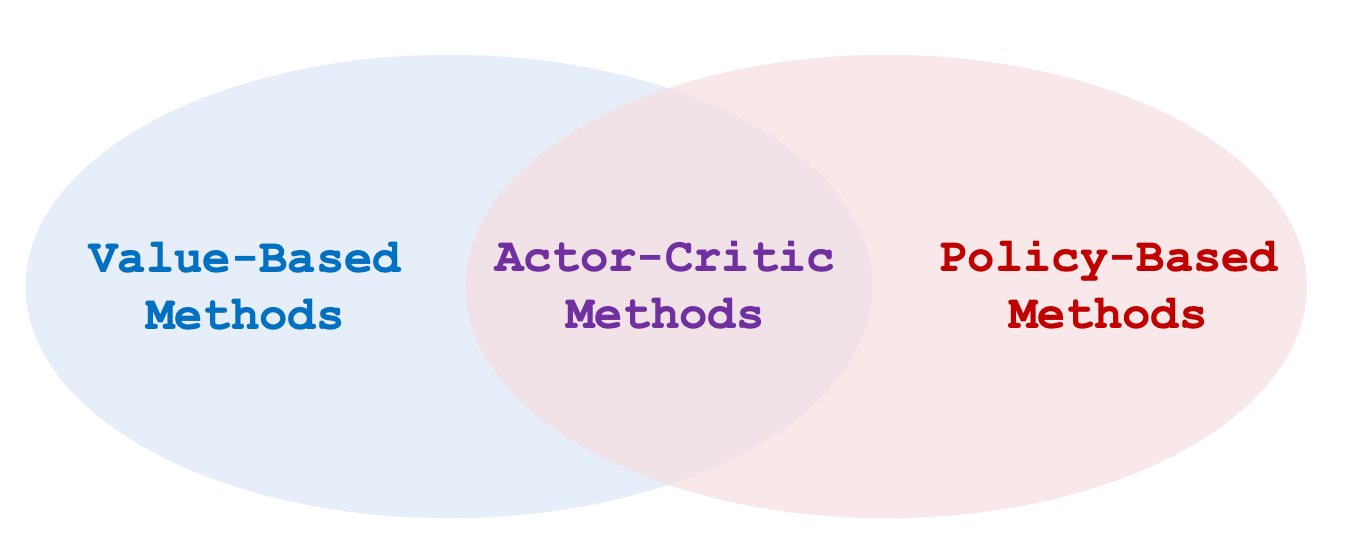### State - Value Function Approximation

$$V_{\pi}\left(s\right) =\sum_{a \in \mathcal{A}} \pi\left(a \mid s\right) \cdot Q_{\pi}\left(s, a\right) \approx \sum_{a \in \mathcal{A}} \pi\left(a \mid s;\boldsymbol{\theta}\right) \cdot q(s, a;\mathbf{w})$$
$\boldsymbol{\theta}, \mathbf{w}$ 分别为Policy Network, Value Network的参数.

### Policy Network (Actor)

Policy Network近似了策略函数$\pi(a\mid s)$, 继续沿用我们之前讲过的结构吧: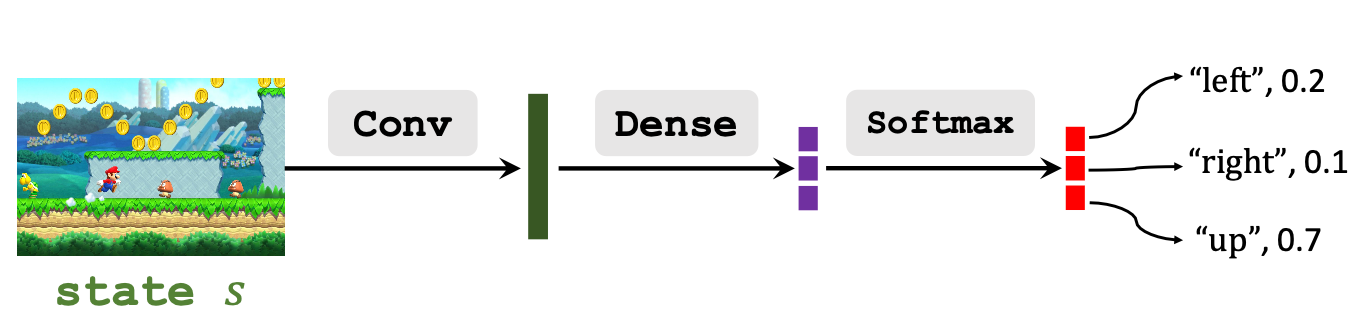Policy Network的输出是在状态$s$ 下选择各个动作$a$ 的概率, $\sum_{a\in \mathcal{A}}\pi(a \mid s; \boldsymbol{\theta})=1$.

### Value Network (Critic)

Value Network近似了动作价值函数$Q_\pi(s, a)$, 根据$s$ 对所有的$a$ 打分.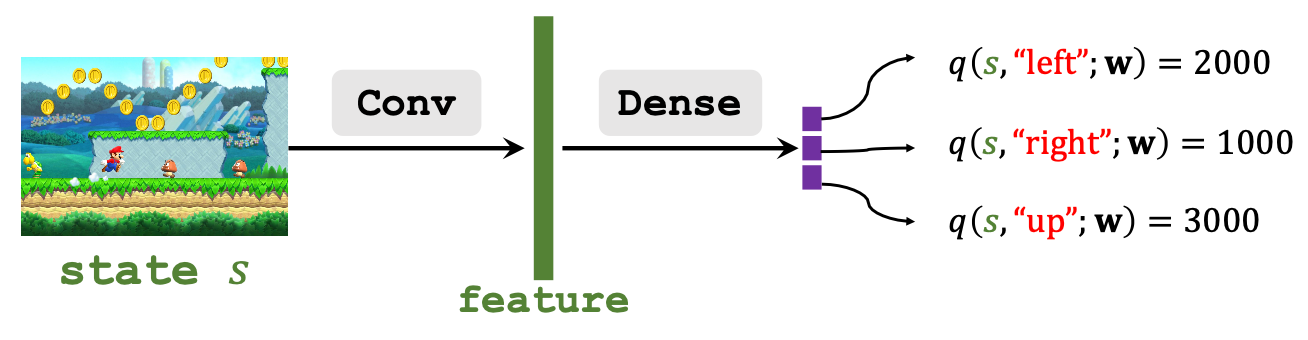Value Network的输出是在状态$s$ 下各动作$a$ 的打分.

### Actor Critic

Policy Network像演员一样, 根据策略做出相应的动作, 而Value Network像评委一样, 评价演员的动作好坏: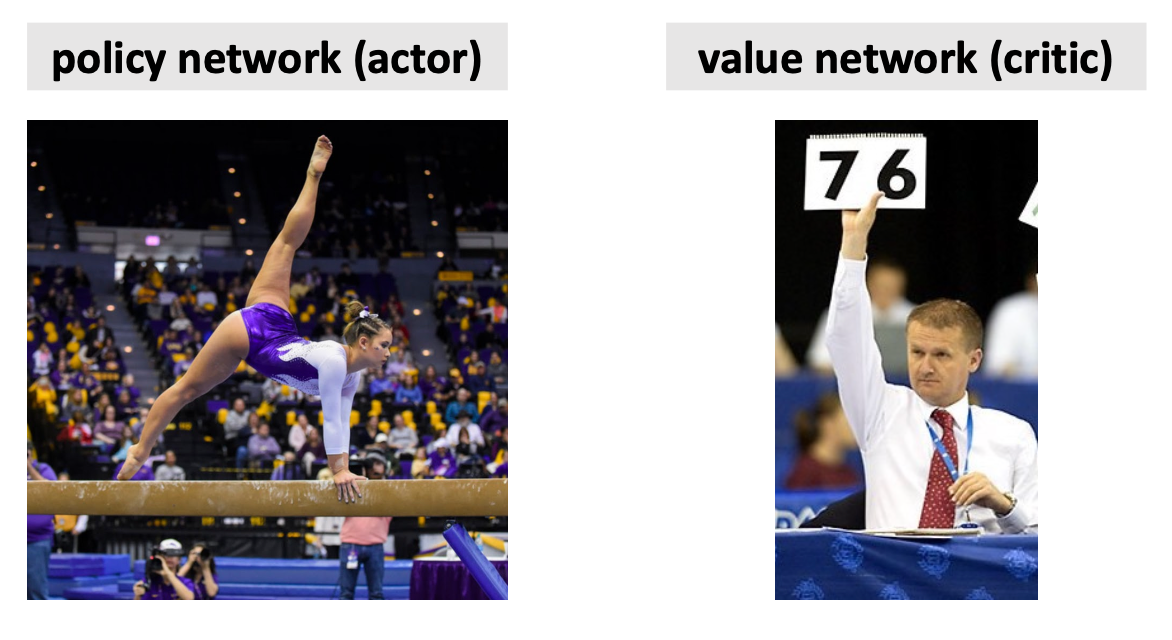### Train the Neural Network

$$V(s; \boldsymbol{\theta}, \mathbf{w}) = \sum_{a \in \mathcal{A}} \pi\left(a \mid s;\boldsymbol{\theta}\right) \cdot q(s, a;\mathbf{w})$$

• 更新Policy Network $\pi\left(a \mid s;\boldsymbol{\theta}\right)$ 是为了让状态价值$V(s; \boldsymbol{\theta}, \mathbf{w})$ 更大, 令演员得到的平均分更高. 所以Policy Network学习时, 依赖的价值是有监督的由Value Network提供的.
• 更新Value Network $q(s, a;\mathbf{w})$ 是为了对演员动作的打分更为精准, 从而更好估计Return. 所以Value Network学习时, 依赖的奖励是有监督的由环境提供的.

Actor观察状态$s$ 后, 做出动作$a$, Critic根据$s$ 和$a$, 给出打分$q$. 然后环境状态转移, 同时给予奖励$r$. Critic从环境奖励中吸取经验改进自己, 演员也根据评委的意见改进自己, 然后如此循环: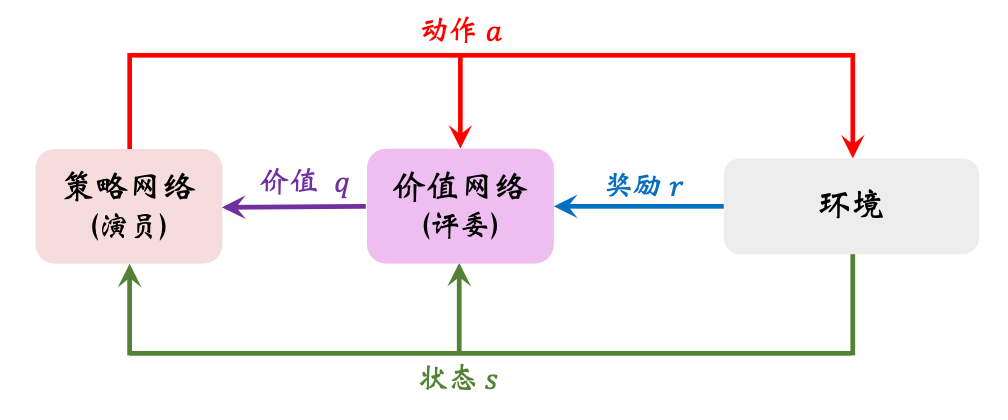• 为了让评委越来越好, 评委根据$s, a, r$ 来改进自己的打分$q$. 可以让评委比较相邻两次的打分$q_t, q_{t+1}, r_t$, 使用TD算法更新参数.

• 为了让演员越来越好, 演员根据$q$ 来改进自己在不同$s$ 下选择$a$ 的能力. 如果裁判很辣鸡, $q$ 就会不准确, 演员为了迎合评委的口味, 自身也会跑偏.

1. 观察状态$s_t$, 并从$\pi\left(\cdot \mid s_t;\boldsymbol{\theta}_t\right)$ 中随机抽样得到动作$a_t$.
2. 令Agent做出动作$a_t$, 同时得到新的环境状态$s_{t+1}$ 和奖励$r_t$.
3. 从$\pi\left(\cdot \mid s_{t+1};\boldsymbol{\theta}_t\right)$ 随机采样出假设要做出的动作$\tilde{a}_{t+1}$, 但并不执行$\tilde{a}_{t+1}$.
4. 为了使用TD算法, 计算两次Value Network的输出$q_t = q(s_t, a_t;\mathbf{w}_t), q_{t+1}=q(s_{t+1}, \tilde{a}_{t+1}; \mathbf{w}_t)$.
5. 计算TD Error, $\delta_t=q_t - (r_t + \gamma\cdot q_{t+1})$, $r_t + \gamma\cdot q_{t+1}$ 即为TD Target.
6. 计算Value Network的导数$\mathbf{d}_{w, t}=\left.\frac{\partial q\left(s_{t}, a_{t} ; \mathbf{w}\right)}{\partial \mathbf{w}}\right|_{\mathbf{w}=\mathbf{w}_{t}}$
7. 用TD算法梯度下降更新Value Network, $\mathbf{w}_{t+1} = \mathbf{w}_t - \alpha \cdot \delta \cdot \mathbf{d}_{w, t}$.
8. 计算Policy Network的导数$\mathbf{d}_{\theta, t}=\left.\frac{\partial \log \pi\left(a_{t} \mid s_{t}, \boldsymbol{\theta}\right)}{\partial \boldsymbol{\theta}}\right|_{\boldsymbol{\theta}=\boldsymbol{\theta}_{t}}$.
9. 用策略梯度上升来更新Policy Network, $\boldsymbol{\theta}_{t+1}=\boldsymbol{\theta}_t + \beta \cdot q_t \cdot \mathbf{d}_{\theta, t}$.

## AlphaGo!

AlphaGO在2016年击败了世界围棋冠军李世石, 这在当时是一件非常轰动的事情, 各大媒体更是把AI吹上天了.

### Go Game

• State: 按照黑白二子在棋盘上的位置来区分, 它的状态可以是$19 \times 19 \times 2$ 的张量, 其值全部为0或1, 用来表示黑 / 白子是否在该位置有子. 实际上AlphaGo使用了更大的状态张量$19 \times 19 \times 48$来存储其他信息.
• Action: 状态空间$\mathcal{A}$ 自然就为棋盘上的361个位置, 即$\mathcal{A} = \set{1, 2, 3 \dots, 361}$.

### High Level Ideas

1. 用Behavior Cloning来初始化训练Policy Network. 其实它是监督学习, 从人类的对战中学习到.
2. 用Reinforcement Learning的策略梯度进一步训练Policy Network, 让两个Policy Network互相对战.
3. 训练完Policy Network后再训练Value Network.

### Policy Network

#### State

AlphaGo有多个版本, 采用的State不一样.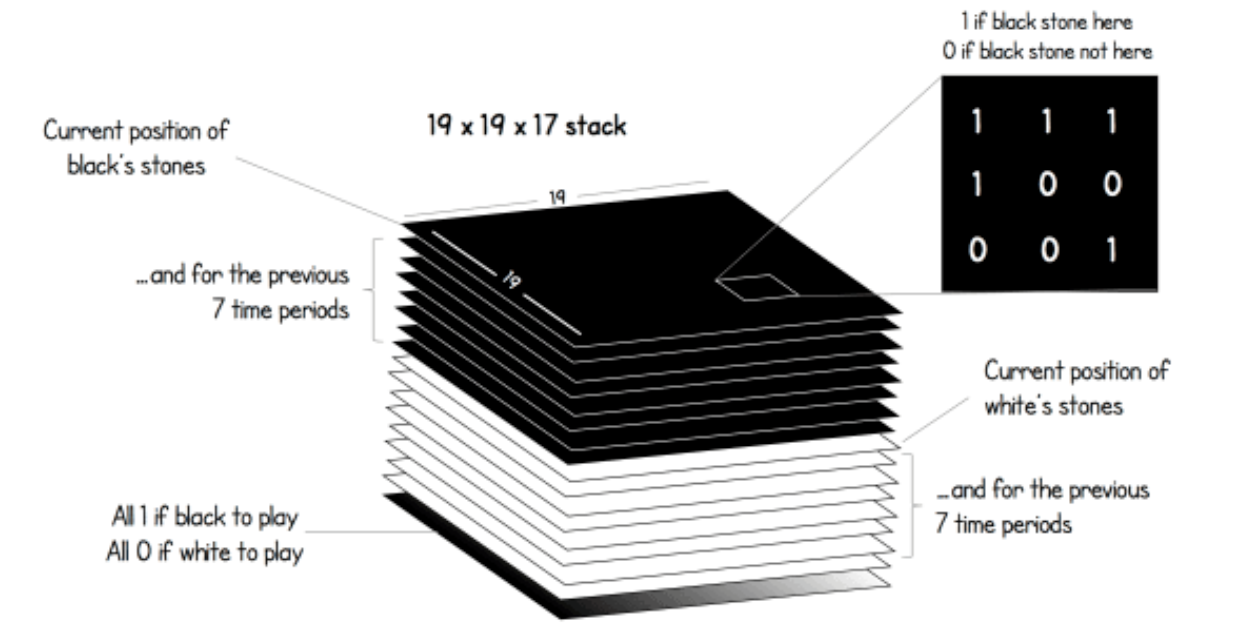• $19 \times 19$ 描述的棋盘的所有位置, 共有17个$19 \times 19$的矩阵.

• 把当前黑子的位置, 和之前7步黑子的位置, 用8个$19 \times\ 19$ 的矩阵来表示.

• 把当前白子的位置, 和之前7步白子的位置, 用8个$19 \times\ 19$ 的矩阵来表示.

• 最后一个矩阵, 也就是图中最下面那个矩阵, 是黑白子指示矩阵, 如果该黑子走了则矩阵全是1, 白子走全是0.

#### Policy Network Architecture

AlphaGo Zero的策略网络结构是这样的: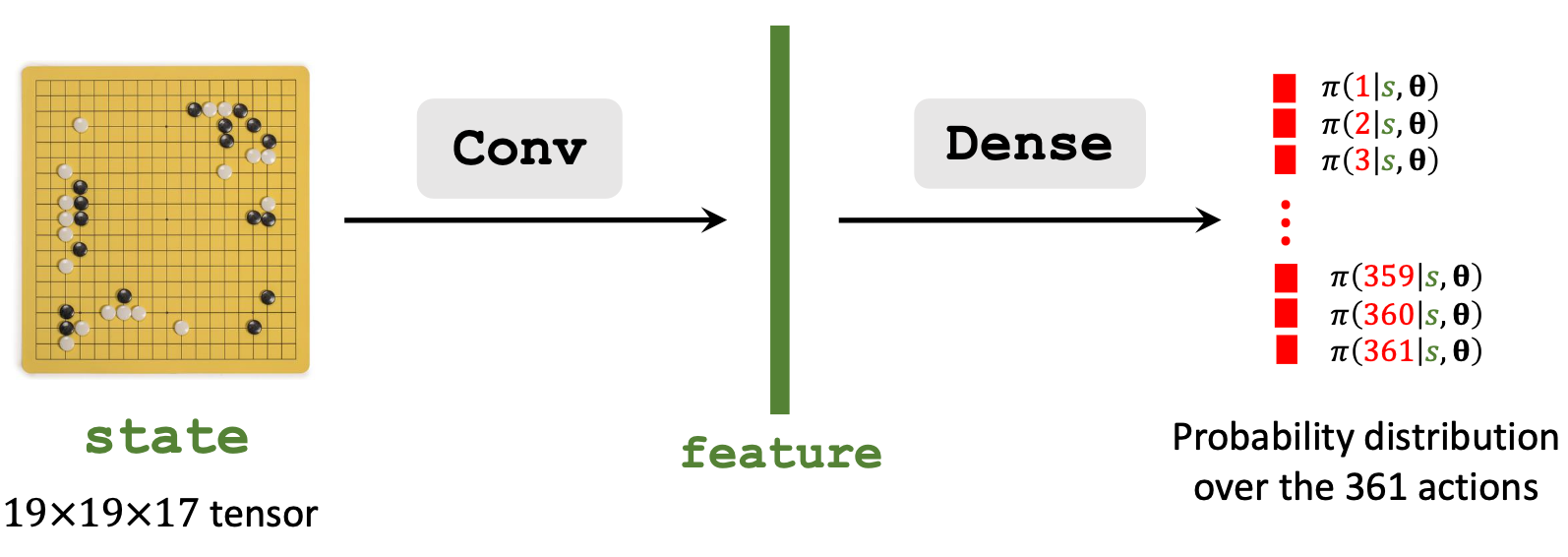AlphaGo初号机的策略网络结构就比较简单了: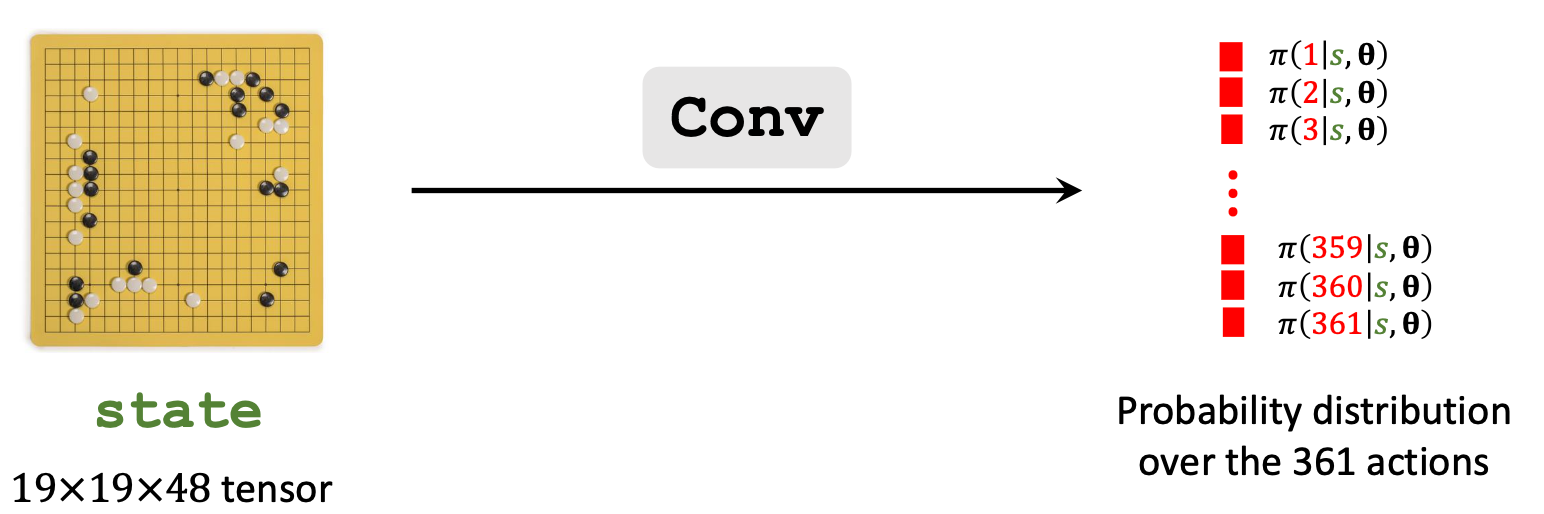#### Behavior Cloning

AlphaGo初号机使用了Behavior Cloning.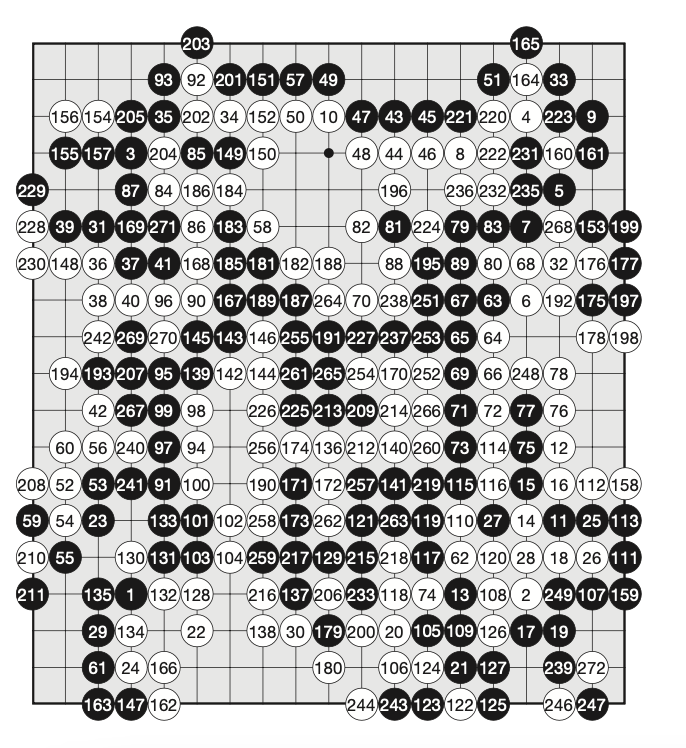Behavior Cloning不是强化学习, 而是一种模仿学习(Imitation Learning), 这种方法与奖励无关. 下面来在场景下来介绍一下它的执行流程:

1. 观察State $s_t$.
2. 用策略网络针对$s_t$ 做个决策: $\mathbf{p}_{t}=\left[\pi\left(1 \mid s_{t}, \boldsymbol{\theta}\right), \cdots, \pi\left(361 \mid s_{t}, \boldsymbol{\theta}\right)\right] \in(0,1)^{361}$.
3. 假设下一步人类玩家做出的动作$a_t^\star=281$, 同时将其编码为One hot标签$y_t \in \set{0, 1}^{361}$.
4. 对$y_t, \mathbf{p}_t$ 用CrossEntropy作为Loss, 梯度下降更新策略网络参数.

Behavior Cloning后AlphaGo已经能碾压围棋小白了, 为啥呢? 大概率是因为$s_t$ 在AlphaGo看过的棋局里面出现过.

#### Train Policy Network Using Policy Gradient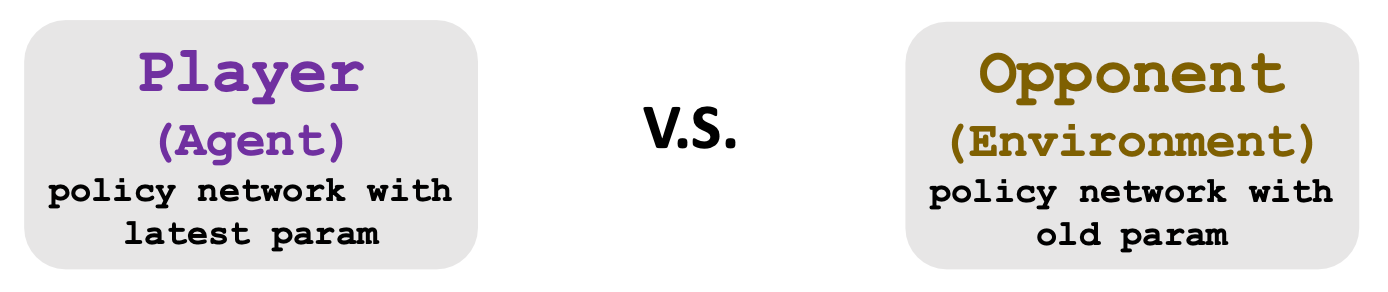#### Reward

• $r_1 = r_2 = r_3 = \cdots = r_{T-1}=0$.
• $r_T = + 1$ (Winner).
• $r_T = -1$ (Loser).

1. 让两个策略网络相互博弈, Agent所使用的策略网络每一把都更新一次参数.
2. 一局打完后会获得一个迹$s_1, a_1, s_2, a_2, \cdots, s_T, a_T$, 以及输赢所对应的$u_T$.
3. 知道这一把的结果和回报后, 求出每一把策略梯度总和$\mathbf{g}_{\theta}=\sum_{t=1}^{T} \frac{\partial \log \pi\left(a_{t} \mid s_{t}, \boldsymbol{\theta}\right)}{\partial \boldsymbol{\theta}} \cdot u_{t}$.
4. 策略梯度上升 $\boldsymbol{\theta} \leftarrow \boldsymbol{\theta} + \beta \cdot \mathbf{g}_\theta$.

### Value Network

1. 仍然是让两个策略网络互相打, 还是赢了的回报为+1, 输了的为-1. 不更新策略网络的参数.
2. 我们希望观测到的回报和价值网络预测的价值相同, 所以使用MSE作为损失函数优化, 即$L=\sum_{t=1}^{T} \frac{1}{2}\left[v\left(s_{t} ; \mathbf{w}\right)-u_{t}\right]^{2}$.
3. 用梯度下降优化价值网络参数, $\mathbf{w} \leftarrow \mathbf{w} - \alpha \cdot \frac{\partial L}{\partial \mathbf{w}}$.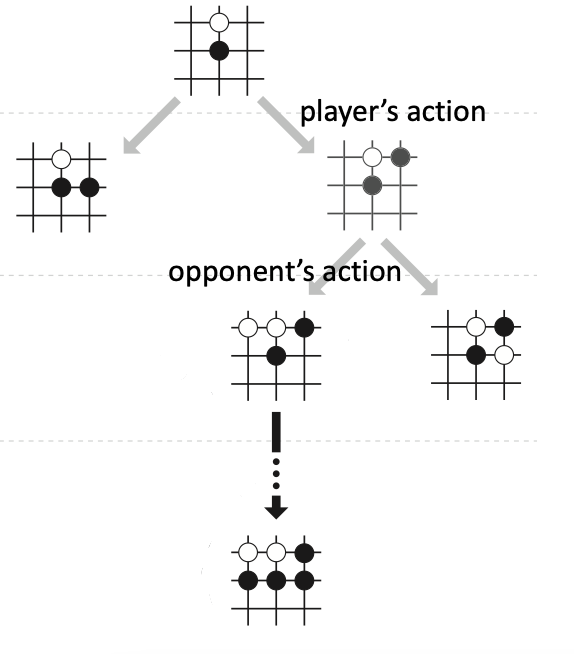• 根据策略网络给出的动作好坏筛选一些好的动作, 随机选择一个动作$a$.
• 让策略网络根据选择的$a$ 做自我博弈, 直到游戏结束, 看看输赢.
• 如此反复, 直到所有好的动作都被穷举完.
• 每个动作$a$ 都应该对应着一个打分, 每回选择最高分数的作为真正要执行的动作.

MCTS以上述四个过程为原型执行.

#### Step 1: Selection

$$\operatorname{score}(a) = Q(a) + \eta \cdot \frac{\pi(a \mid s_t; \boldsymbol{\theta})}{1 + N(a)}$$

• $Q(a)$: 它是一张搜索记录计算出来的动作价值表, 后面会解释.
• $\pi(a \mid s_t; \boldsymbol{\theta})$: 策略网络根据$s_t$ 选择的动作$a$的概率.
• $N(a)$: 对于$s_t$, $a$ 已经被探索过的次数, 这样可以减少$a$ 被过多探测的次数.
• $\eta$: 超参数, 作为加权系数.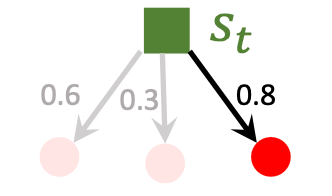#### Step 2: Expansion

$$a_t^\prime \sim \pi(\cdot \mid s_t^\prime; \boldsymbol{\theta})$$
$s_t^\prime$ 指的是对手视角的状态.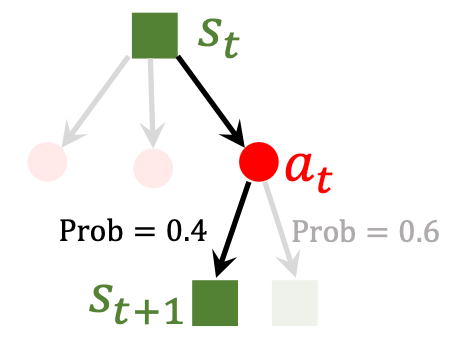#### Step 3: Evaluation

• Player: $a_k \sim \pi(\cdot \mid s_k; \boldsymbol{\theta})$.
• Opponent: $a_k^\prime \sim \pi(\cdot \mid s_k^\prime; \boldsymbol{\theta})$.
• 当分出胜负时, 赢了则$r_T=+1$, 输了$r_T=-1$. 这种奖励可以一定程度评价$s_{t+1}$ 的好坏. 应该想办法令赢了增加状态$s_{t+1}$ 的评分, 反之降低.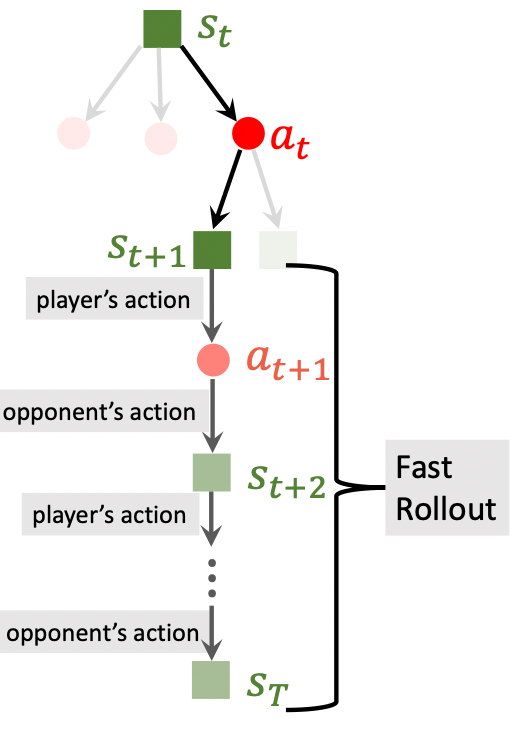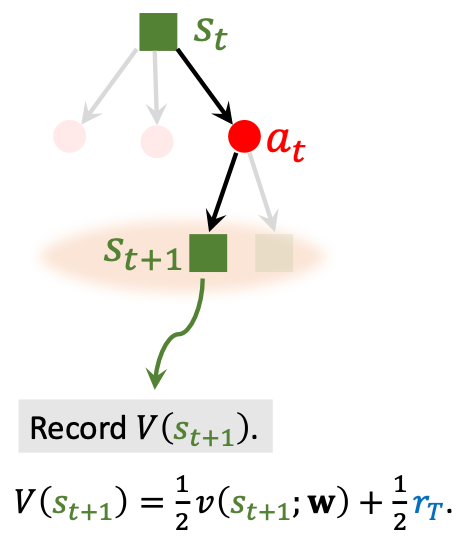$s_{t+1}$ 越好, $V(s_{t+1})$ 就越高, 如果往回追溯, $a_t$ 动作就越好.

#### Step 4: Backup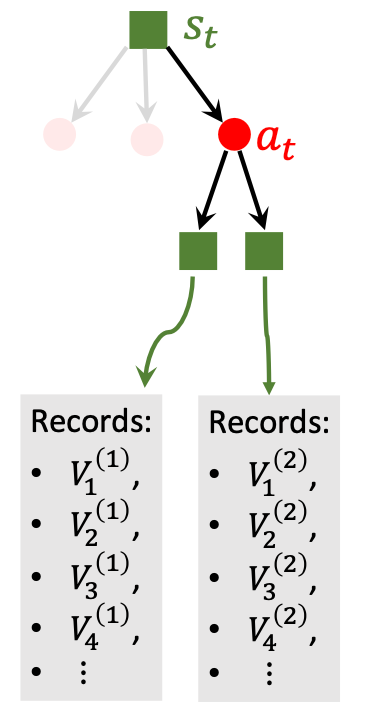$$Q(a_t)= \operatorname{mean}(\text{recorded } V\text{‘s})$$

$$\operatorname{score}(a) = Q(a) + \eta \cdot \frac{\pi(a \mid s_t; \boldsymbol{\theta})}{1 + N(a)}$$

• 刚开始时$Q(a)$ 都为0, 所以基本上依赖后一项, 也就是策略网络给出的打分来判断动作$a$ 好坏.
• 随着模拟次数增加, 策略网络觉得越好的动作就越有可能被选中, 即$N(a)$ 变大, 第二项缩小, 后期会主要依赖动作价值$Q(a)$ 来评价动作好坏.

#### Decision Making after MCTS

AlphaGo做决策很简单, 就是选择被选中次数最多的动作作为实际所选择的动作$a_t$:
$$a_{t}=\underset{a}{\operatorname{argmax}} N(a)$$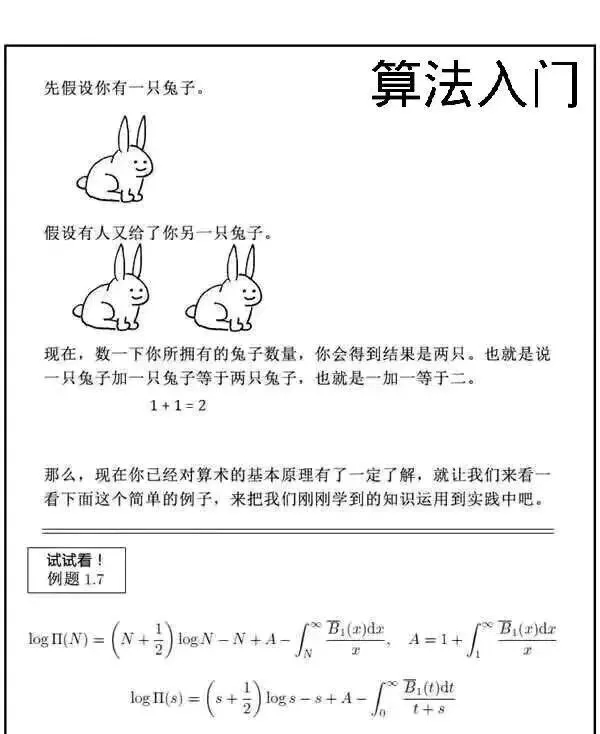上一篇T5: Exploring the Limits of Transfer Learning with a Unified Text-to-Text Transformer

2022-04-22Hexo博客迁移
Hexo博客迁移本文记录一次带主题的Hexo博客迁移过程, 从Win上迁移到MacOS. 前置依赖首先, 需要在MacOS上装Node.js和Git的环境, 网上有大把大把的教程, 这里就不再多说了, 自行搜索即可. 必要文件把Window
2022-03-17
目录